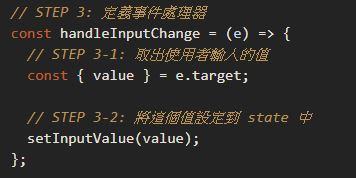#DAY 10
4
Modern Web

## [Day 10 - 網速換算器] 換算起來吧 - 資料綁定與組件拆分

• [複習] `setState` 的使用
• [複習] 條件渲染
• [New] 綁定資料在表單元素上
• [New] 將 JSX 拆成多個組件

• 輸入 `Mbps` 自動轉換成 `MB/s`
• 根據輸入的數字不同，最下方會呈現不同的樣式和文字說明## 取得使用者輸入的資料

``````const SpeedConverter = () => {
return (
{/* ... */}
<div className="card-body">
<div className="converter">
<div className="flex-1">
<div className="converter-title">Set</div>
{/* ⚠️ 取得使用者輸入的內容 */}
<input type="number" className="input-number" min="0" />
</div>
{/* ... */}
</div>
</div>
{/* ... */}
);
};
``````

### 綁定 onChange 事件

``````{/* ... */}
<div className="flex-1">
<div className="converter-title">Set</div>
<input
type="number"
onChange={() => console.log("onChange")}
className="input-number"
min="0"
/>
</div>
{/* ... */}
``````### 透過 useState 讓 React 明白資料的變化``````// STEP 1：取出 useState 這個方法
const { useState } = React;

const SpeedConverter = () => {
// STEP 2: 定義 state，取得預設值為 0 的 inputValue 和修改該狀態的 setInputValue 方法
const [inputValue, setInputValue] = useState(0);

// STEP 3: 定義事件處理器
const handleInputChange = (e) => {
// STEP 3-1: 取出使用者輸入的值
const { value } = e.target;

// STEP 3-2: 將這個值設定到 state 中
setInputValue(value);
};

return (
<div className="container">
<div className="card-body">
<div className="unit-control">
<div className="unit">Mbps</div>
<span className="exchange-icon fa-fw fa-stack">
<i className="far fa-circle fa-stack-2x" />
<i className="fas fa-exchange-alt fa-stack-1x" />
</span>
<div className="unit">Mb/s</div>
</div>
<div className="converter">
<div className="flex-1">
<div className="converter-title">Set</div>
{/* STEP 4: 把事件處理器綁定進去，並且把 value 帶入 */}
<input
type="number"
onChange={handleInputChange}
value={inputValue}
className="input-number"
min="0"
/>
</div>
<span
className="angle-icon fa-2x"
style={{
marginTop: 30,
}}
>
<i className="fas fa-angle-right" />
</span>
<div className="text-right flex-1">
<div className="converter-title">Show</div>
{/* STEP 5: 把使用者輸入的值顯示於畫面上 */}
<input
className="input-number text-right"
type="text"
value={inputValue}
disabled
/>
</div>
</div>
</div>
<div className="card-footer">FAST</div>
</div>
);
};

ReactDOM.render(<SpeedConverter />, document.getElementById('root'));
``````

• Step 1：從 React 中取出 `useState` 這個方法
• Step 2：使用 `useState()` 定義 `inputValue` 這個資料狀態（state）預設值為 0，並取得更新狀態的 `setInputValue` 這個方法
• Step 3：定義事件處理器（`handleInputChange`），透過 `e.target.value` 可以取得使用者輸入的內容，這裡透過解構賦值的方式把 `value` 取出來，接著透過 `setInputValue` 更新在 React 中資料狀態
• Step 4：把 `handleInputChange` 放到 `onChange` 的事件處理器中；把對話框 `value` 的值等同於 React 中 `inputValue` 的值
• Step 5：透過 `value={inputValue}` 把使用者輸入的 `inputValue` 顯示於畫面上。## 進行網速單位換算``````{/* STEP 5: 把使用者輸入的值顯示於畫面上 */}
<input
className="input-number text-right"
type="text"
value={inputValue / 8}
disabled
/>
``````

## 拼積木嘍 - React 組件的拆分React 組件的拆分非常簡單，其實你早就會了。舉例來說，現在想要把 `<div className="unit-control">...</div>` 的這個區塊拆成一個獨立的組件：``````const UnitControl = () => (
<div className="unit-control">
<div className="unit">Mbps</div>
<span className="exchange-icon fa-fw fa-stack">
<i className="far fa-circle fa-stack-2x" />
<i className="fas fa-exchange-alt fa-stack-1x" />
</span>
<div className="unit">Mb/s</div>
</div>
);
````````````const { useState } = React;

const UnitControl = () => (
<div className="unit-control">
<div className="unit">Mbps</div>
<span className="exchange-icon fa-fw fa-stack">
<i className="far fa-circle fa-stack-2x" />
<i className="fas fa-exchange-alt fa-stack-1x" />
</span>
<div className="unit">Mb/s</div>
</div>
);

const CardFooter = () => {
return <div className="card-footer">FAST</div>;
};

const SpeedConverter = () => {
const [inputValue, setInputValue] = useState(0);

const handleInputChange = (e) => {
const { value } = e.target;
setInputValue(value);
};

return (
<div className="container">
<div className="card-body">
<UnitControl />
<div className="converter">
<div className="flex-1">
<div className="converter-title">Set</div>
<input
type="number"
onChange={handleInputChange}
value={inputValue}
className="input-number"
min="0"
/>
</div>
<span
className="angle-icon fa-2x"
style={{
marginTop: 30,
}}
>
<i className="fas fa-angle-right" />
</span>
<div className="text-right flex-1">
<div className="converter-title">Show</div>
<input
className="input-number text-right"
type="text"
value={inputValue / 8}
disabled
/>
</div>
</div>
</div>
<CardFooter />
</div>
);
};

ReactDOM.render(<SpeedConverter />, document.getElementById('root'));
``````

## 條件渲染的使用

``````const CardFooter = () => {
// STEP 1：inputValue 是使用者輸入的數值，暫時先設成 30
let inputValue = 30;
// STEP 2：定義 criteria 物件
let criteria;

// STEP 3：根據 inputValue 改變要顯示的內容和背景色
if (!inputValue) {
criteria = {
title: '---',
backgroundColor: '#d3d8e2',
};
} else if (inputValue < 15) {
criteria = {
title: 'SLOW',
backgroundColor: '#ee362d',
};
} else if (inputValue < 40) {
criteria = {
title: 'GOOD',
backgroundColor: '#1b82f1',
};
} else if (inputValue >= 40) {
criteria = {
title: 'FAST',
backgroundColor: '#13d569',
};
}

return (
<div className="card-footer">
Fast
</div>
);
};
``````

``````// STEP 4：把定義好的 criteria 物件帶入 JSX 內
return (
<div
className="card-footer"
style={{
backgroundColor: criteria.backgroundColor
}}
>
{criteria.title}
</div>
);
``````## 參考文章

• Forms @ React Docs - main concepts

### 1 則留言

0Step 3：定義事件處理器（handleInputChange）

const { value } = e.target;

pjchender iT邦新手 5 級 ‧ 2020-01-29 23:12:34 檢舉

``````const text = e.target.value;
``````

``````const value = e.target.value;

// 變成直接把 e.target 這個物件中的 value 取出
// 並同時建立一個名為 value 的變數
const { value } = e.target;
``````

ert60213 iT邦新手 5 級 ‧ 2020-01-30 14:08:54 檢舉

``````const value = e.target;
// e.target 這個物件本身就內含value嗎
``````
pjchender iT邦新手 5 級 ‧ 2020-03-02 14:49:46 檢舉

ert60213 是的，這個物件內本身就含有 value。